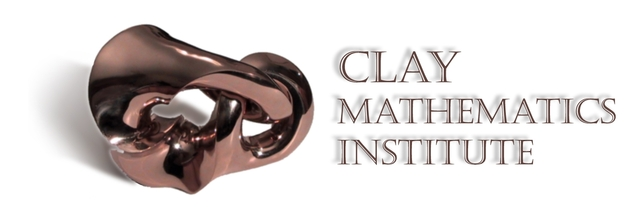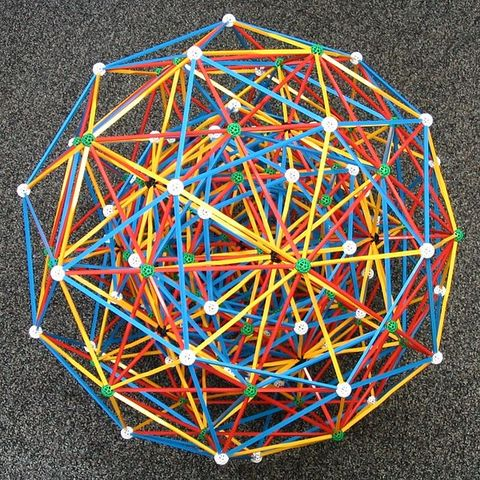• Period: to

• # Kepler Conjecture has been proved."Proof of Kepler's Conjecture by Thomas C. Hales" The Kepler conjecture, named after the 17th-century German astronomer Johannes Kepler, is a mathematical conjecture about sphere packing in three-dimensional Euclidean space.
• # Clay Mathematics Institute proposes the seven Millennium Prize Problems of unsolved important classic mathematical questions."Millenium Prize Problems" The Millennium Prize Problems are seven problems in mathematics that were stated by the Clay Mathematics Institute in 2000. As of July 2011, six of the problems remain unsolved. A correct solution to any of the problems results in a US\$1,000,000 prize (sometimes called a Millennium Prize) being awarded by the institute.
• # Modularity Theorem (Taniyama–Shimura conjecture) has been proved."Proof of Fermat's Last theorem" It postulates that elliptic curves over the field of rational numbers are related to modular forms. Andrew Wiles proved the modularity theorem for semistable elliptic curves, which was enough to imply Fermat's last theorem, and Christophe Breuil, Brian Conrad, Fred Diamond, and Richard Taylor extended his techniques to prove the full modularity theorem in 2001.
• # AKS Primality Test"AKS Primality Article" The AKS primality test (also known as Agrawal–Kayal–Saxena primality test and cyclotomic AKS test) is a deterministic primality-proving algorithm created and published by three Indian Institute of Technology Kanpur computer scientists, Manindra Agrawal, Neeraj Kayal, and Nitin Saxena, on August 6, 2002, in a paper titled "PRIMES is in P"
• # Pi at its 1.24 trillion places"Professor Yasumasa Kanada broke his own world record." Kanada and a team of researchers set a new world record by calculating the value of pi to 1.24 trillion places.
• # Poincaré conjecture has been proved"Grigori Perelman's Poincaré Conjecture's Proof" Poincaré conjecture is a theorem about the characterization of the three-dimensional sphere (3-sphere), which is the hypersphere that bounds the unit ball in four-dimensional space.
• # Catalan's Conjecture has been proved."Preda Mihăilescu's Proof of Catalan's Conjecture" To understand the conjecture, notice that 23 and 32 are two powers of natural numbers, whose values 8 and 9 respectively are consecutive. The conjecture states
• # E8 Mapping Was Introduced"The E8 Mathematics" Mathematicians have mapped the inner workings of one of the most complicated structures ever studied: the object known as the exceptional Lie group E8. This achievement is significant both as an advance in basic knowledge and because of the many connections between E8 and other areas, including string theory and geometry.